# `sklearn.decomposition`.PCA¶

class `sklearn.decomposition.``PCA`(n_components=None, copy=True, whiten=False, svd_solver=’auto’, tol=0.0, iterated_power=’auto’, random_state=None)[source]

Principal component analysis (PCA)

Linear dimensionality reduction using Singular Value Decomposition of the data to project it to a lower dimensional space.

It uses the LAPACK implementation of the full SVD or a randomized truncated SVD by the method of Halko et al. 2009, depending on the shape of the input data and the number of components to extract.

It can also use the scipy.sparse.linalg ARPACK implementation of the truncated SVD.

Notice that this class does not support sparse input. See `TruncatedSVD` for an alternative with sparse data.

Read more in the User Guide.

Parameters: n_components : int, float, None or string Number of components to keep. if n_components is not set all components are kept: ```n_components == min(n_samples, n_features) ``` if n_components == ‘mle’ and svd_solver == ‘full’, Minka’s MLE is used to guess the dimension if `0 < n_components < 1` and svd_solver == ‘full’, select the number of components such that the amount of variance that needs to be explained is greater than the percentage specified by n_components n_components cannot be equal to n_features for svd_solver == ‘arpack’. copy : bool (default True) If False, data passed to fit are overwritten and running fit(X).transform(X) will not yield the expected results, use fit_transform(X) instead. whiten : bool, optional (default False) When True (False by default) the components_ vectors are multiplied by the square root of n_samples and then divided by the singular values to ensure uncorrelated outputs with unit component-wise variances. Whitening will remove some information from the transformed signal (the relative variance scales of the components) but can sometime improve the predictive accuracy of the downstream estimators by making their data respect some hard-wired assumptions. svd_solver : string {‘auto’, ‘full’, ‘arpack’, ‘randomized’} auto : the solver is selected by a default policy based on X.shape and n_components: if the input data is larger than 500x500 and the number of components to extract is lower than 80% of the smallest dimension of the data, then the more efficient ‘randomized’ method is enabled. Otherwise the exact full SVD is computed and optionally truncated afterwards. full : run exact full SVD calling the standard LAPACK solver via scipy.linalg.svd and select the components by postprocessing arpack : run SVD truncated to n_components calling ARPACK solver via scipy.sparse.linalg.svds. It requires strictly 0 < n_components < X.shape randomized : run randomized SVD by the method of Halko et al. New in version 0.18.0. tol : float >= 0, optional (default .0) Tolerance for singular values computed by svd_solver == ‘arpack’. New in version 0.18.0. iterated_power : int >= 0, or ‘auto’, (default ‘auto’) Number of iterations for the power method computed by svd_solver == ‘randomized’. New in version 0.18.0. random_state : int, RandomState instance or None, optional (default None) If int, random_state is the seed used by the random number generator; If RandomState instance, random_state is the random number generator; If None, the random number generator is the RandomState instance used by np.random. Used when `svd_solver` == ‘arpack’ or ‘randomized’. New in version 0.18.0. components_ : array, shape (n_components, n_features) Principal axes in feature space, representing the directions of maximum variance in the data. The components are sorted by `explained_variance_`. explained_variance_ : array, shape (n_components,) The amount of variance explained by each of the selected components. Equal to n_components largest eigenvalues of the covariance matrix of X. New in version 0.18. explained_variance_ratio_ : array, shape (n_components,) Percentage of variance explained by each of the selected components. If `n_components` is not set then all components are stored and the sum of explained variances is equal to 1.0. singular_values_ : array, shape (n_components,) The singular values corresponding to each of the selected components. The singular values are equal to the 2-norms of the `n_components` variables in the lower-dimensional space. mean_ : array, shape (n_features,) Per-feature empirical mean, estimated from the training set. Equal to X.mean(axis=0). n_components_ : int The estimated number of components. When n_components is set to ‘mle’ or a number between 0 and 1 (with svd_solver == ‘full’) this number is estimated from input data. Otherwise it equals the parameter n_components, or n_features if n_components is None. noise_variance_ : float The estimated noise covariance following the Probabilistic PCA model from Tipping and Bishop 1999. See “Pattern Recognition and Machine Learning” by C. Bishop, 12.2.1 p. 574 or http://www.miketipping.com/papers/met-mppca.pdf. It is required to computed the estimated data covariance and score samples. Equal to the average of (min(n_features, n_samples) - n_components) smallest eigenvalues of the covariance matrix of X.

References

For n_components == ‘mle’, this class uses the method of Thomas P. Minka: Automatic Choice of Dimensionality for PCA. NIPS 2000: 598-604

Implements the probabilistic PCA model from: M. Tipping and C. Bishop, Probabilistic Principal Component Analysis, Journal of the Royal Statistical Society, Series B, 61, Part 3, pp. 611-622 via the score and score_samples methods. See http://www.miketipping.com/papers/met-mppca.pdf

For svd_solver == ‘arpack’, refer to scipy.sparse.linalg.svds.

For svd_solver == ‘randomized’, see: Finding structure with randomness: Stochastic algorithms for constructing approximate matrix decompositions Halko, et al., 2009 (arXiv:909) A randomized algorithm for the decomposition of matrices Per-Gunnar Martinsson, Vladimir Rokhlin and Mark Tygert

Examples

```>>> import numpy as np
>>> from sklearn.decomposition import PCA
>>> X = np.array([[-1, -1], [-2, -1], [-3, -2], [1, 1], [2, 1], [3, 2]])
>>> pca = PCA(n_components=2)
>>> pca.fit(X)
PCA(copy=True, iterated_power='auto', n_components=2, random_state=None,
svd_solver='auto', tol=0.0, whiten=False)
>>> print(pca.explained_variance_ratio_)
[ 0.99244...  0.00755...]
>>> print(pca.singular_values_)
[ 6.30061...  0.54980...]
```
```>>> pca = PCA(n_components=2, svd_solver='full')
>>> pca.fit(X)
PCA(copy=True, iterated_power='auto', n_components=2, random_state=None,
svd_solver='full', tol=0.0, whiten=False)
>>> print(pca.explained_variance_ratio_)
[ 0.99244...  0.00755...]
>>> print(pca.singular_values_)
[ 6.30061...  0.54980...]
```
```>>> pca = PCA(n_components=1, svd_solver='arpack')
>>> pca.fit(X)
PCA(copy=True, iterated_power='auto', n_components=1, random_state=None,
svd_solver='arpack', tol=0.0, whiten=False)
>>> print(pca.explained_variance_ratio_)
[ 0.99244...]
>>> print(pca.singular_values_)
[ 6.30061...]
```

Methods

 `fit`(X[, y]) Fit the model with X. `fit_transform`(X[, y]) Fit the model with X and apply the dimensionality reduction on X. `get_covariance`() Compute data covariance with the generative model. `get_params`([deep]) Get parameters for this estimator. `get_precision`() Compute data precision matrix with the generative model. `inverse_transform`(X) Transform data back to its original space. `score`(X[, y]) Return the average log-likelihood of all samples. `score_samples`(X) Return the log-likelihood of each sample. `set_params`(**params) Set the parameters of this estimator. `transform`(X) Apply dimensionality reduction to X.
`__init__`(n_components=None, copy=True, whiten=False, svd_solver=’auto’, tol=0.0, iterated_power=’auto’, random_state=None)[source]
`fit`(X, y=None)[source]

Fit the model with X.

Parameters: X : array-like, shape (n_samples, n_features) Training data, where n_samples in the number of samples and n_features is the number of features. y : Ignored. self : object Returns the instance itself.
`fit_transform`(X, y=None)[source]

Fit the model with X and apply the dimensionality reduction on X.

Parameters: X : array-like, shape (n_samples, n_features) Training data, where n_samples is the number of samples and n_features is the number of features. y : Ignored. X_new : array-like, shape (n_samples, n_components)
`get_covariance`()[source]

Compute data covariance with the generative model.

`cov = components_.T * S**2 * components_ + sigma2 * eye(n_features)` where S**2 contains the explained variances, and sigma2 contains the noise variances.

Returns: cov : array, shape=(n_features, n_features) Estimated covariance of data.
`get_params`(deep=True)[source]

Get parameters for this estimator.

Parameters: deep : boolean, optional If True, will return the parameters for this estimator and contained subobjects that are estimators. params : mapping of string to any Parameter names mapped to their values.
`get_precision`()[source]

Compute data precision matrix with the generative model.

Equals the inverse of the covariance but computed with the matrix inversion lemma for efficiency.

Returns: precision : array, shape=(n_features, n_features) Estimated precision of data.
`inverse_transform`(X)[source]

Transform data back to its original space.

In other words, return an input X_original whose transform would be X.

Parameters: X : array-like, shape (n_samples, n_components) New data, where n_samples is the number of samples and n_components is the number of components. X_original array-like, shape (n_samples, n_features) :

Notes

If whitening is enabled, inverse_transform will compute the exact inverse operation, which includes reversing whitening.

`score`(X, y=None)[source]

Return the average log-likelihood of all samples.

See. “Pattern Recognition and Machine Learning” by C. Bishop, 12.2.1 p. 574 or http://www.miketipping.com/papers/met-mppca.pdf

Parameters: X : array, shape(n_samples, n_features) The data. y : Ignored. ll : float Average log-likelihood of the samples under the current model
`score_samples`(X)[source]

Return the log-likelihood of each sample.

See. “Pattern Recognition and Machine Learning” by C. Bishop, 12.2.1 p. 574 or http://www.miketipping.com/papers/met-mppca.pdf

Parameters: X : array, shape(n_samples, n_features) The data. ll : array, shape (n_samples,) Log-likelihood of each sample under the current model
`set_params`(**params)[source]

Set the parameters of this estimator.

The method works on simple estimators as well as on nested objects (such as pipelines). The latter have parameters of the form `<component>__<parameter>` so that it’s possible to update each component of a nested object.

Returns: self :
`transform`(X)[source]

Apply dimensionality reduction to X.

X is projected on the first principal components previously extracted from a training set.

Parameters: X : array-like, shape (n_samples, n_features) New data, where n_samples is the number of samples and n_features is the number of features. X_new : array-like, shape (n_samples, n_components)

Examples

```>>> import numpy as np
>>> from sklearn.decomposition import IncrementalPCA
>>> X = np.array([[-1, -1], [-2, -1], [-3, -2], [1, 1], [2, 1], [3, 2]])
>>> ipca = IncrementalPCA(n_components=2, batch_size=3)
>>> ipca.fit(X)
IncrementalPCA(batch_size=3, copy=True, n_components=2, whiten=False)
>>> ipca.transform(X)
```

## Examples using `sklearn.decomposition.PCA`¶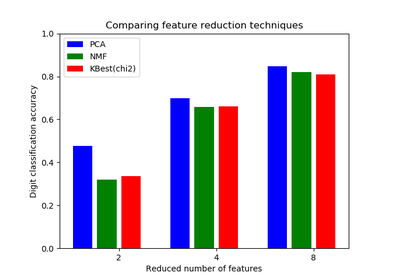Selecting dimensionality reduction with Pipeline and GridSearchCV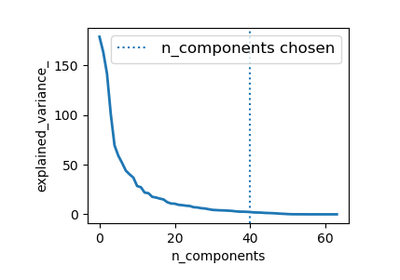Pipelining: chaining a PCA and a logistic regressionConcatenating multiple feature extraction methods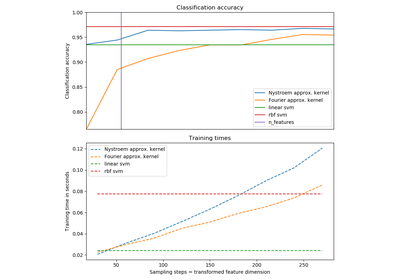Explicit feature map approximation for RBF kernels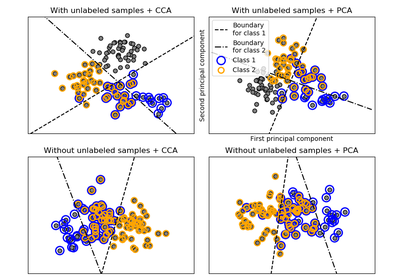Multilabel classificationFaces recognition example using eigenfaces and SVMs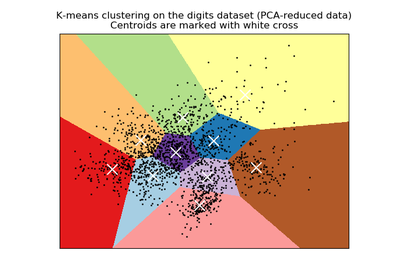A demo of K-Means clustering on the handwritten digits data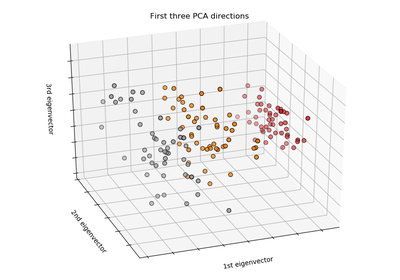The Iris Dataset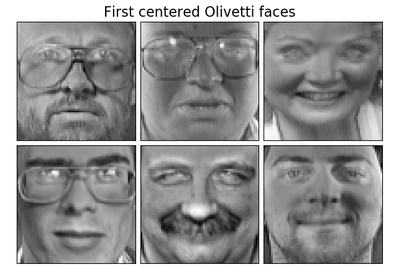Faces dataset decompositions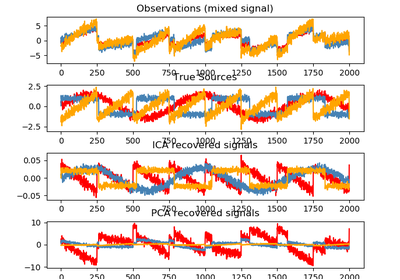Blind source separation using FastICA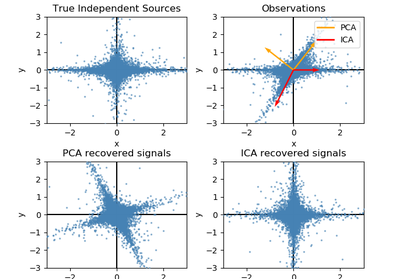FastICA on 2D point clouds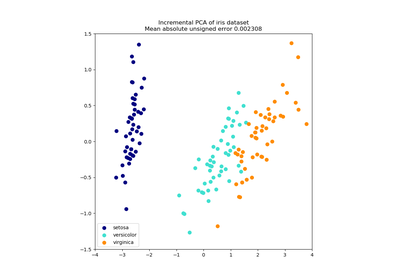Incremental PCA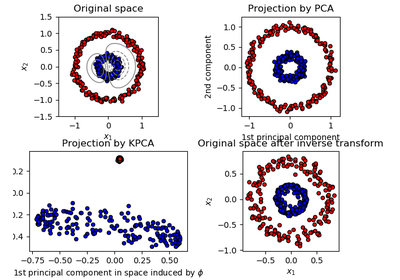Kernel PCA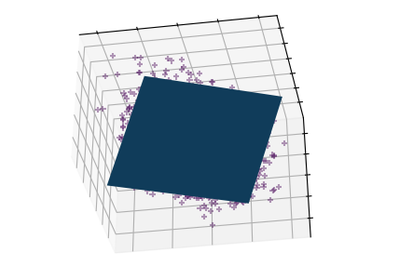Principal components analysis (PCA)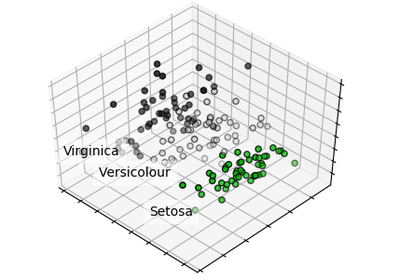PCA example with Iris Data-set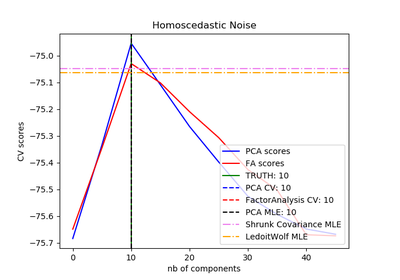Model selection with Probabilistic PCA and Factor Analysis (FA)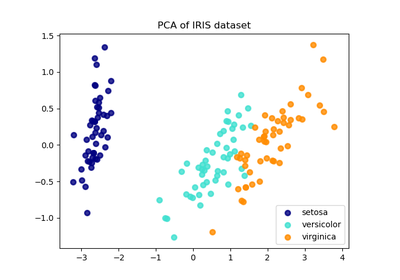Comparison of LDA and PCA 2D projection of Iris dataset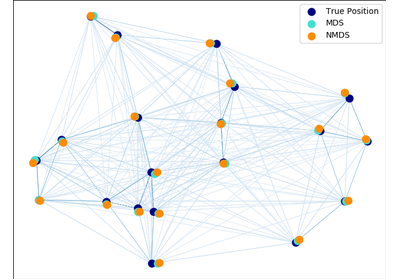Multi-dimensional scaling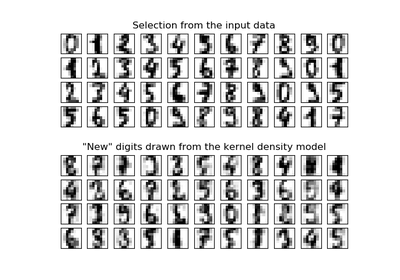Kernel Density Estimation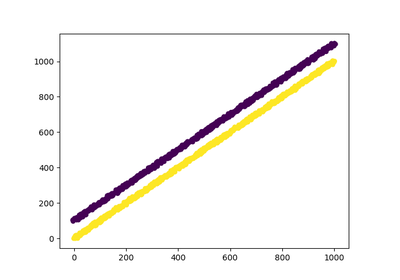Using FunctionTransformer to select columns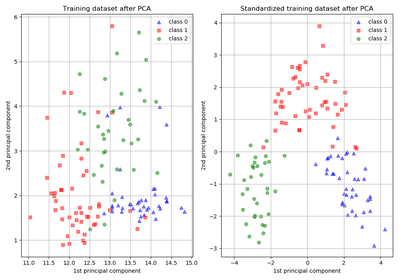Importance of Feature Scaling